##### Actions

The value of a function, defined on the unit disc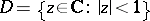, at a boundary point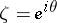, equal to the limitof the functionon the set of points of the radius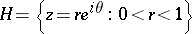leading to the point. The term "radial boundary value" is sometimes used in a generalized sense for functionsgiven on arbitrary (including multi-dimensional) domains, whereis taken to be the set of points of a normal (or its analogue) to the boundary ofleading to the boundary point. For example, in the case of a bi-disc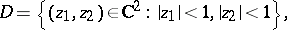as the radial boundary value at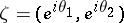one takes the limit